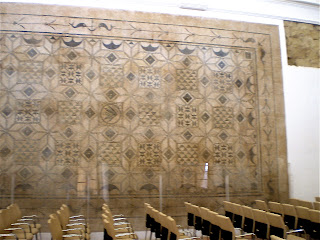## Thursday, December 23, 2010

### Hyperbolic geometry in Roman mosaics?

The Real Alcazar in Cordoba, Spain contains a wonderful collection of mosaics from the Roman period. The patterns used are geometrical in nature and I have already shown some examples of the impossible Thiery-figure in one of the other blog posts. One large mosaic caught my attention because it contained some images I did not really expect to see.The mosaic displayed against the wallClose-up of the border of the mosaic

A close-up of the mosaic shows these images as the edge of the mosaic whose edges are semicircles.
Zooming in on one individual image we see clearly that the top of the black region is a semi-circle and the bottom is made up of two smaller semi-circles.

Hyperbolic geometry is one possible non-Euclidean geometry (another is spherical geometry). There are several different ways to model this space. One of those is the so-called "Upper half-space Model". Instead of using the whole plane like we do in Euclidean geometry, we only use the upper half of the plane (as suggested by the name).

In Euclidean geometry we draw images of polygons using straight line segments. These segments are the shortest possible curve between two points. In hyperbolic geometry the sides of polygons are made up of vertical straight lines or semi-circles that meet the bottom of the half-space in a right angle.
In other words, the black object shown above is a hyperbolic triangle!

It takes a little getting used to, but in hyperbolic space the angles are smaller than the ones we see in Euclidean geometry. This triangle has three very small angles and the sum of the angles of a triangle is definitely not 180 degrees in this geometry. In hyperbolic geometry the sum of the angles in a triangle is actually always strictly smaller than 180 degrees.